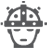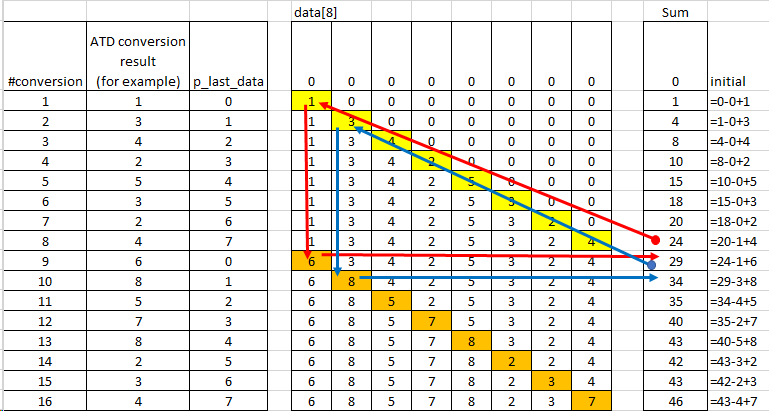cancel
Showing results for
Did you mean:

2,402 ViewsContributor I

Hello,

I am working on moving average code for ADC. I got 8 samples from ADC and as per my knowledge, while moving average: (1+2+.....8)/8 will be first average, (2+3+....9)/8 will be second ...

Is this a correct way of implementation?

If anyone has a sample code, then please provide.

Regards,

7 Replies
1,167 ViewsNXP TechSupport

Hi,

really fast idea to make moving average filter, probably, I hope, I am not wrong....not tested....

unsigned int new_conversion_processing(unsigned int new_data)
{
static unsigned int data = { 0,0,0,0,0,0,0,0 }; // data set
static unsigned char p_oldiest_data = 0;          // "pointer" to data to be changed
static unsigned int  sum;                                // sum of the data set

sum = sum-data[p_oldiest_data] + new_data;  // calculate a new sum by removing the oldest data from it

data[p_oldiest_data] = new_data;                    // replace the oldest value by the the newest one in the buffer

p_oldiest_data = (p_oldiest_data==7) ? 0 :(p_oldiest_data++);

return(sum>>3);                                             // return average value (=sum/8)
}

Process flow description:For such calculations, it is good to be XGATE used to store data in continuous background process and you then only share the result to CPU for next processing. Of course, this is a little bit different story.

Best regards,

1,167 ViewsSenior Contributor II

I know of two algorithms that might satisfy your requirements, although I do not know what you need to accomplish. Without knowing the processor you wish to use, I can't provide code. The implementations could vary widely - I've implemented the second algorithm one way in an 8-bit MCU, and in a very different way in a DSP.

The first algorithm is what you describe - It's called a Boxcar filter. It is a special case of a FIR filter (finite-impulse response filter), where the coefficients are all the same. It will produce a true average over the most recent N samples. It is computationally inexpensive: For memory, it just uses a table for the most recent N samples and the running average, and the math requires only an add and a subtract and maybe a shift.

The second is called an exponential filter, and is a type of IIR filter (infinite-impulse response filter). It is different than a true average in that the most recent value has the most weight in the final result, the second most recent has less weight, and on down. It's response is identical to an electrical RC filter. For memory, it only needs the new sample and the previous output. It can be implemented as two multiplies and an add, or it can be implemented as a subtract, a shift and an add.

If you describe the problem better, we may be able to help you better.

Mark

1,167 ViewsContributor I

Hey,

Sorry for not providing all the necessary information.

I am using Mc9s12XEP100 microcontroller, and ADC is on chip, having 12 bit resolution.  I want to implement it in a embedded C code. So, how these concepts can be applied?

Well thank you for your explaination.

Regards,

1,167 ViewsSenior Contributor II

Since I don't know the purpose of your filter, I can't suggest which one would be better. If you are using it for a control application, the exponential filter would likely be better (faster step-response and smoother transitions). If you are using it for numerical analysis, the boxcar filter may be better. If you need the filter for decimation, both would work, but I would lean toward the boxcar.

All of my code is written in assembler, for the DSP563xx of for the HC08/S08. But since the exponential filter is so simple to implement in C, here is some code (not verified for syntax):

```static float coefficient;        // 0 through 1 for how much filtering to do

/*
This function implements an exponential filter
on a sample, returning the filtered value.
*/
float filter( float sample )
{
static float result;

result = coefficient*sample + (1-coefficient)*result
return result;
}

/*
This function sets the strength of the filter, and
has a valid range of zero to one. A one will not filter
at all, returning the sample unmodified, and a zero
will freeze    the result at its current value.
If the new coefficient is outside of the valid range,
then it will remain unmodified.
*/
void setCoefficient ( float newCoefficient )
{
if ( newCoefficient >= 0  ) && ( newCoefficient <= 1  )
coefficient = newCoefficient;
}```

If you would like the filter to respond similarly to an average of eight samples, then you would want to set the coefficient to one-eighth (1/8, 0r 0.125). With the exponential filter, the filter coefficient can be changed on-the-fly.

If you need a true average, then that code would need a static array of the most recent samples, operated as a ring-buffer. You would also need the ring-buffer index to be static. Rather than adding all samples and the dividing by the number of samples, you should keep a static copy of the first result, and then simply subtract-out the oldest sample and add-in the newest sample with each new sample.

Hope that helps.

Mark

1,167 ViewsContributor I

Hey Mark,

Yes, I am using it for numerical analysis. So, from the methods you have suggested will opt for Boxcar filter.

Well, Thank you so much for such a detailed answer.

Regards,

1,167 ViewsNXP Employee

Could you please specify the product that you are using (NXP part number)?

Thank you,

Paulina

1,167 ViewsContributor I

Hey,

Sorry for not providing all the necessary information.

I am using Mc9s12XEP100 microcontroller, and ADC is on chip, having 12 bit resolution.

Regards,